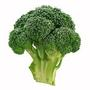1. Forum
2. >
3. Topic: Welsh
4. >
5. "Symiau rhannu"

# "Symiaurhannu"

## Translation:Division problems

April 24, 2016•19

Division problems would be "problemau rhannu" and that doesn't really mean anything. I think this answer needs to be changed.

The sort of basic arithmetic division sums you would do in primary school - '12 divided by 3 is 4', 'what is 10 divided by 2.5? and so on.I see, thanks. "Division sums" sounds like a contradiction in terms in American English!

Mod
Plus
•2173

What would be more natural in American English? Would you say 'Math Division' or 'Math Division Sums'? Any other suggestion we could add?If I understand ibisc correctly, maybe just "division problems"? Does the term refer to the exercises that kids are doing to learn to divide or to division as a school subject? If it's the latter, I'm not sure that there's a natural US equivalent.

Mod
Plus
•2173

It's the first concept, 'division problems' sounds like a good translation.drewissimo33 is correct in his comments above. I'm from Canada and I'm equally confused by this strange "Division sums". For us, "sums" ONLY ever means the result of addition (or, possibly, subtraction if we're talking about adding negative numbers). It definitely seems like the word "sums" is also equivalent to the word "problems" or "questions" in Wales?

Mod
Plus
•2173

It is really an informal way of saying problems or questions relating to division.

For older children in schools both 'problemau rhannu' and 'cwestiynau rhannu' are used to describe 'division problems' and 'division questions'

symiau rhannu is understood as the same as both.

symiau on its own (sums) is an informal way of talking about arithmetic

It is easily understood by pupils in Welsh medium schools, while the more formal rhifyddeg is hard for pupils to understand because it's not part of everyday speech.

Duo has added a note on this Britishism in the section notes.Now children, we're going to do some maths, some math (in America), some mathematics (quite formal to children), some maths problems, some arithmetic, some addition and subtraction, some adding and subtracting (young children, non-technical), some multiplication and division, some multiplying and dividing (young children, non-technical). Grab out your books. Maths is fun! (I agree, the only meaning of "sum" or "sums" is addition.)•1394

"Division sums" certainly is a contradiction. A sum is the result of the operation "addition", a quotient is the result of the operation "division". A division can never end up in a sum!'Sums' (plural) can mean any simple arithmetic - so we might do multiplication sums, subtraction sums, division sums, addition sums, even though the end result of an addition is 'the sum' (singular), of a multiplication it is the 'product' and for a division, the 'quotient'. Can't remember any specific term for the result of a subtraction! Children in days gone by were often told 'be quiet and get on with your sums'.